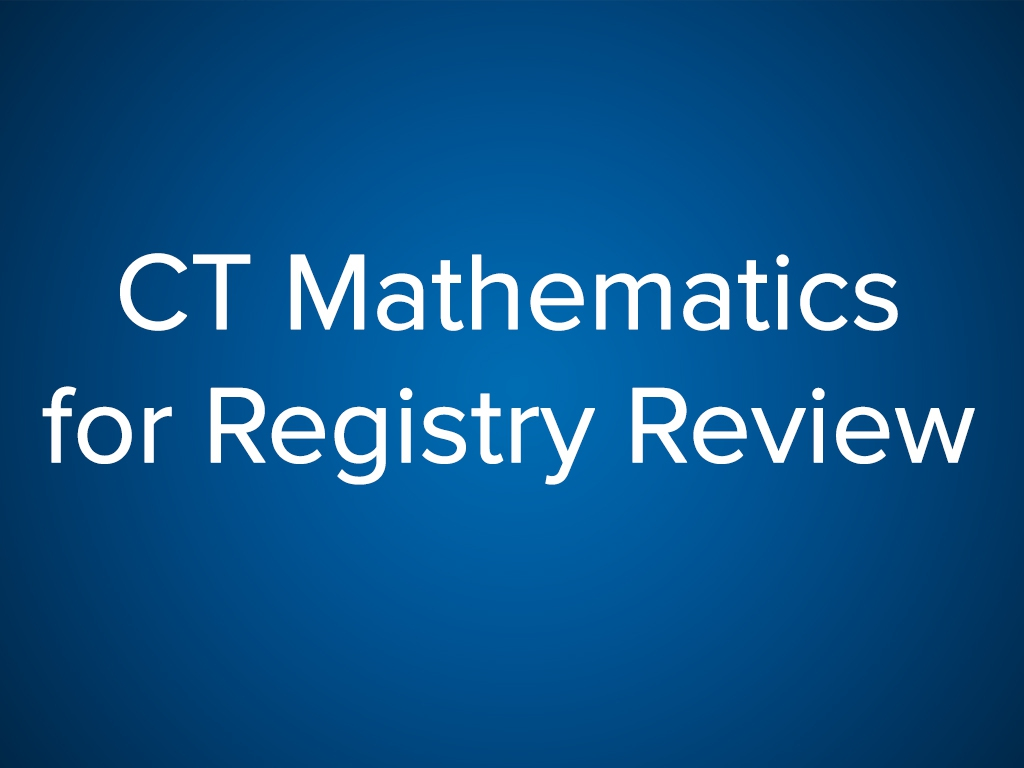CT Mathematics for Registry Review (CE ARRT)

Course Format: Online Video
Course Credit Type: CE ARRT
Course Credit: 1.00
Rewards Points Earned: 125
Course Category: *Imaging, CT
Accreditation: This course is accredited by the Association of Vascular and Interventional Radiographers (AVIR) an approved
Course Cost: \$17.99 | Included in All-Access Pass
Mobile Friendly: Yes
Course Release Date: June 16, 2018
Course Expiration Date: June 16, 2019
Program Summary:

In a Power Point format, seventeen (17) of the most important relationships that have their basis in mathematics are discussed. Included in the discussion are the sharing of the relationships illustrated by what each entity in each equation means. The algebra used to solve most equations is discussed in details including all math calculations and equalities. Finally, appropriate examples are used in problem solving activities to illustrate and demonstrate the relevancy of the individual mathematical equation. The learner will observe that there are many mathematical relationships that can be used to explain certain CT fundamentals.

Objectives
With 80% proficiency, as a result of this activity, participants will be able to perform the following:
1. Recognize the appropriate equation for the application considered.
2. State the definition of the values considered on each side of the equal sign in every equation.
3. Recognize the applicability of the solution realized when proper mathematical computations are completed.
4. Understand the underlying concepts demonstrated with appropriate mathematical applications.
Outline
1. The mathematics presented include the equations and statements as follows:
1. HEAT UNITS: mA x seconds x kVp x 1.41
2. PIXEL SIZE: FOV / MATRIX
3. VOXEL SIZE: PIXEL SIZE (SQUARED) X SLICE THICKNESS
4. ANATOMY COVERED: SLICE THICKNESS X # OF SLICES
5. BEAM WIDTH: SLICE THICKNESS X # OF DETECTORS UTILIZED
6. SDCT PITCH: TABLE TRAVEL DURING TUBE ROTATION / SLICE THICKNESS
7. MDCT PITCH: TABLE TRAVEL DURING TUBE ROTATION / BEAM WIDTH
8. CT NUMBER EQUATION
9. TESTING FOR LINEARITY
10. TESTING FOR UNIFORMITY
11. LINEAR ATTENUATION COEFFICIENT
12. THE WAVE EQUATION
13. SDCT SCAN COVERAGE
14. MDCT SCAN COVERAGE
15. CALCULATING EFFECTIVE DOSE IN mSv
16. INTERPOLATION
17. CALCULATING WL & WW
2. Each equation is broken down explaining the values to be used on each side of the equal sign in order to accurately calculate a solution.
3. The relevancy of the equations and their solutions as they apply to CT physics and fundamentals is explained in detail.
You need to log in or create a user account before you can enroll.